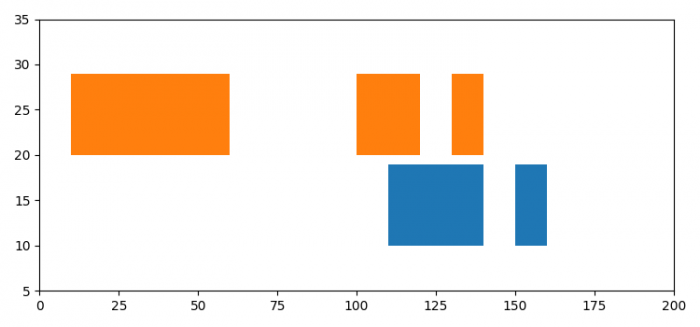# How to get a Gantt plot using matplotlib?

Gantt charts are widely used in project planning to show the project schedule. It's a type of bar chart that lists the tasks on the vertical axis and the time intervals on the horizontal axis. The width of the horizontal bars in the graph shows the duration of each activity.

To plot a Gantt chart in matplotlib, we can use the broken_barh() method.

## Steps

• Set the figure size and adjust the padding between and around the subplots.

• Create a figure and a set of subplots.

• Plot a horizontal sequence of rectangles.

• Set the y and x limits of the axes.

• To display the figure, use show() method.

## Example

import matplotlib.pyplot as plt

# Set the figure size
plt.rcParams["figure.figsize"] = [7.50, 3.50]
plt.rcParams["figure.autolayout"] = True

# Figure and set of subplots
fig, ax = plt.subplots()

# Horizontal sequence of rectangles
ax.broken_barh([(110, 30), (150, 10)], (10, 9), facecolors='tab:blue')
ax.broken_barh([(10, 50), (100, 20), (130, 10)], (20, 9), facecolors='tab:orange')

# ylim and xlim of the axes
ax.set_ylim(5, 35)
ax.set_xlim(0, 200)

# Show the plot
plt.show()

## Output

It will produce the following output −Updated on: 01-Feb-2022

462 Views